# GSEB Solutions Class 11 Physics Chapter 15 Waves

Gujarat Board GSEB Textbook Solutions Class 11 Physics Chapter 15 Waves Textbook Questions and Answers.

## Gujarat Board Textbook Solutions Class 11 Physics Chapter 15 Waves

### GSEB Class 11 Physics Waves Text Book Questions and Answers

Question 1.
A string of mass 2.50 kg is under a tension of 200 N. The length of the stretched string is 20.0 m. If the transverse jerk is struck at one end of the string, how long does the disturbance take to reach the other end?
Here, Tension, T = 200 N
length of string, l = 20.0 m
mass of string, M = 2.50 kg
mass per unit length of the string,
∴ We know that the velocity of transverse wave is given by.
v = $$\sqrt{\frac{T}{m}}$$ = $$\sqrt{\frac{200}{0.125}}$$ = 40 ms-1
Let t = time taken by the transverse jerk to reach from one end to the other end of the string.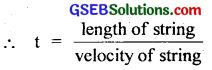= $$\frac{l}{v}$$ = $$\frac{20}{40}$$ = 0.5 s.Question 2.
A stone dropped from the top of a tower of height 300 m high splashes into the water of a pond near the base of the tower. When is the splash heard at the top given that the speed of sound in air is 340 ms-1? (g = 9.8 ms-2)
Here, Initial velocity of stone,
u = 0
a = g = 9.8 ms-2
height of Tower, h = 300 m
velocity of sound in air, v = 340 ms-1
Let t1 = time taken by the stone to fall down
t2 = time taken by the sound to travel from the bottom to the top of tower
If t = time after which splash is heard at the top, then
t = t1 + t2 …………………….. (i)
Now to find t1 let us use the equation
s = ut + $$\frac{1}{2}$$ at2
Here, s = h, u = 0, a = g, t = t1
∴ h = 0 + $$\frac{1}{2}$$ gt21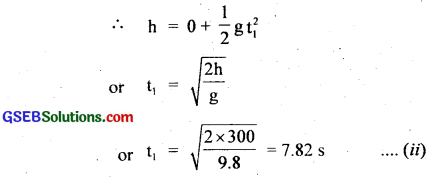To find t2 let us use the formula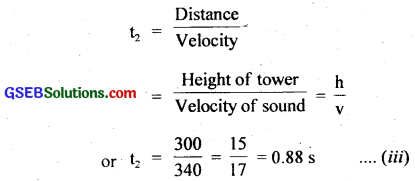∴ From (i), (ii) and (iii), we get
t = 7.82 + 0.88 = 8.7 s.

Question 3.
A steel wire has a length of 12.0 m and a mass of 2.10 kg. What should be the tension in the wire so that speed of a transverse wave on the wire equals the speed of sound in dry air at 20°C = 343
Here,
length of steel wire, l = 12.0 m
Mass of steel wire, M = 2.10 kg
Let T = tension in the wire = ?
If m = mass per unit length of the wire, then
m = $$\frac{M}{l}$$ = $$\frac{2.10}{12.0}$$ = 0.175 kg m-1
V = Speed of transverse waves on the wire
= speed of sound in dry air at 20°C = 343 ms-1 (given)
We know that speed of transverse wave in the wire is
v = $$\sqrt{\frac{T}{m}}$$
or v2 = $$\frac{T}{m}$$
or T = mv2
= 0.175 × (343)2
= 20588.6 kg ms-2
= 2.05886 × 104N (∵ 1 kg ms-2 = 1N)
= 2.06 × 104N.Question 4.
Use the formula v = $$\sqrt{\frac{\gamma \mathbf{P}}{\rho}}$$ to explain why the speed of sound in air:
(a) is independent of pressure.
(b) increases with temperature.
(c) inicreases with humidity.
(a) The formula for velocity of sound in air is
v = $$\sqrt{\frac{\gamma \mathbf{P}}{\rho}}$$ ………………………. (1)
where γ = constant for given gas i.e. air
ρ = density of the gas
According to gas equation, we know that PV = RT
or P = $$\frac{RT}{V}$$ ………………………… (2)
∴ From (1) and (2), we get
v = $$\sqrt{\frac{\gamma \mathrm{RT}}{\rho \mathrm{V}}}$$ = $$\sqrt{\frac{\gamma \mathrm{RT}}{\rho \mathrm{M}}}$$ …………………….. (3)
where ρV = M = molecular weight of air or gas.
For a given gas, m = constant.
R is also constant.
If T = constant, then from equation (3), we conclude that v is independent of the pressure of air (gas) if temperature remains constant.

Aliter:
According to Boyle’s law, we know that
PV = constant at a constant temperature. ………………………….. (i)
Now volume of gas,
V = $$\frac{M}{ρ}$$ ………………………….. (ii)
∴ From (i) and (ii), we get
$$\frac{PM}{ρ}$$ = constant
since mass of a gas remains constant P
∴ $$\frac{P}{ρ}$$ = constant
γ is always constant for a given gas or air equation, we conclude that velocity of sound in air always remains constant if its temperature is constant.

(b) Effect of temperature:
We know that
PV = RT
or P = $$\frac{RT}{V}$$
Also v = $$\sqrt{\frac{\gamma \mathbf{P}}{\rho}}$$ = $$\sqrt{\frac{\gamma \mathrm{RT}}{\rho \mathrm{V}}}$$ = $$\sqrt{\frac{\gamma \mathrm{RT}}{\mathrm{M}}}$$
where M = ρV = molecular weight of the gas
As γ, R and M are constants, so
v ∝ $$\sqrt{T}$$
i.e. velocity of sound in a gas is directly proportional to the square root of its temperature, hence we conclude that the velocity of sound in air increases with increase in temperature.

(c) Effect of humidity:
Effect of humidity: The presence of water vapours in air changes the density of air, thus the velocity of sound changes with humidity of air.
Let ρm = density of moist air.
ρd = density of dry air.
Vm = velocity of sound in moist air.
Vd = velocity of sound in dry air.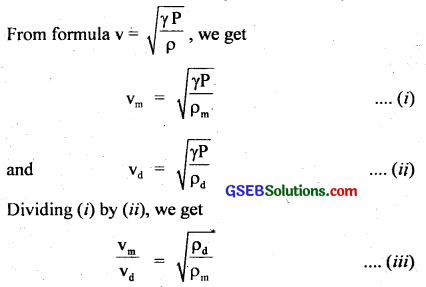Also we know that density of water vapours is less than the density of dry air i.e. dry air is heavier than water vapours as the molecularmass of water is less than that of N2 (28) and O2 (32), so ρm < ρd
or $$\frac{\rho_{\mathrm{d}}}{\rho_{\mathrm{m}}}$$ > 1 ………………………. (iv)
∴ From (iii) and (iv), we get
$$\frac{\mathbf{V}_{\mathrm{m}}}{\mathbf{v}_{\mathrm{d}}}$$ > 1
or vm > vd
i.e. velocity of souind in air increases with humidity. i.e. velocity of moist air is greater than the velocity of sound in dry air. That is why sound travels faster on a rainy day than on a dry day.Question 5.
You have learnt that a travelling wave ¡n one dimension is represented by a function y = f(x, t) where x and t must appear in the combination x – vt, x + vt i.e. y = F(x ± Vt). Is the converse true? Examine if the following functions for y can possibly represent a travelling wave:
(a) (x – vt)2
(b) log $$\left[\frac{x+v t}{x_{0}}\right]$$
(c) $$\frac{1}{(x+vt)}$$
No, the converse ¡s not true.
The basic requirement for a wave function to represent a travelling wave is that for all values of x and t, the wave function must have a finite value.
Out of the given fùnctions for y, none satisfies this condition, so these functions do not represent a travelling wave.

Question 6.
A bat emits ultrasonic sound of frequency 1000 kHz in air. If the sound meets a water surface, what is the wavelength of
(a) the reflected sound
(b) the transmitted sound? Speed of sound in air is 340 ms-1, and in water = 1486 ms-1.
Here, frequency, v = 1000 kHz
= 1000 × 103 Hz = 106 s-1
Speed of sound in air, vs = 340 ms-1
Speed of sound in water, vw = 1486 ms-1

(a) For reflected sound:
After reflection, the ultrasonic sound again travels in air. If λa be the wavelength of the reflected sound, then using the relation V = vλ, we get
λa = $$\frac{v_{a}}{v}=\frac{340}{10^{6}}$$
= 3.4 × 10-4

(b) For transmitted sound:
The transmitted sound travels through water. If λ1 be the wavelength of the transmitted sound, then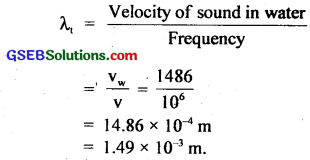Question 7.
A hospital uses an ultrasonic scanner to locate tumours in a tissue. What is the wavelength of sound in the tissue in which the speed of sound is 1.7 kms-1? The operating frequency of the scanner is 4.2 MHz.
Here, speed of sound, v = 1.7 kms-1
= 1.7 × 103 ms-1
Frequency of the scanner, v = 4.2 MHz = 4.2 × 106 Hz
Let λ = wavelength of sound in the tissue = ?
From the relation v = vλ
λ = $$\frac{v}{v}$$
or λ = $$\frac{v}{v}$$ = $$\frac{1.7 \times 10^{3}}{42 \times 10^{6}}$$ = 4.05 × 10-4m
∴ λ = 4.05 × 10-4m = 4.1 × 10-4m.Question 8.
A transverse harmonic wave on a string is described by y(x, t) = 3.0 sin (36t + 0.018x + $$\frac{π}{4}$$)
where x and y are in cm and t in s. The positive direction of x is from left to right.
(a) Is this a travelling wave or a stationary wave? If it is travelling, what are the speed and direction of its propagation?
(b) What are its amplitude and frequency?
(c) What is the initial phase at the origin?
(d) What is the least distance between two successive crests in the wave?
The equation of the given transverse harmonic wave is
y(x, t) = 3.0 sin (36t + 0.018x + $$\frac{π}{4}$$) ………………………. (i)
The standard equation for a travelling wave is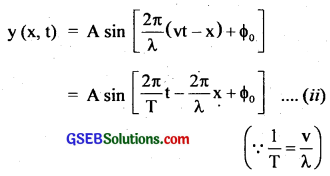(a) Comparing equation (i) and (ii) we see that the equation (i) represents a travelling wave
And $$\frac{2π}{T}$$ = 36
– $$\frac{2π}{λ}$$ = 0.018
or λ = – $$\frac{2π}{0.018}$$ ………………………… (iii)
or 2πv = 36 ………………….. (iv)

Multiplying (iii) and (iv), we get
or 2πvλ = – $$\frac{2 \pi \times 36}{0.018}$$
or vλ = – 2000
or v = – 2000 cms-1
= – 20 ms-1

where v = vλ is the velocity of wave. Here – ve sign shows that the wave travels from right to left.
∴ speed = 20 ms-1
direction = Right to left

(b) A = 3.0 cm
= 3.0 × 10-2m
$$\frac{2π}{T}$$ = 36
v = $$\frac{36}{2π}$$ = $$\frac{36}{2×3.14}$$ = 5.73 Hz

(c) Initial phase, ϕ = $$\frac{π}{4}$$ rad.

(d) The least distance between two successive crests in the wave
= wavelength = λ
= $$\frac{2π}{0.018}$$ = $$\frac{2×3.14}{0.018}$$ = 348.9 cm
= 3.489 m = 3.49 m = 3.5 m.Question 9.
For the wave described in exercise 15.8 plot the displacement (y) versus time (t) graphs for x = 0,2 and 4 cm. What are the shape of these graphs? In which aspects does oscillatory motion in travelling wave differ from one point to another: amplitude, frequency or phase?
Here, the given wave is:
y(x, t) = 3.0 sin (36t + 0.018x + $$\frac{π}{4}$$) …………………….. (i)
Let y1, y2 and y1 be the displacements of the wave for x = 0, 2 and 4 cm.
∴ y1(0, t) = 3.0 sin (36t + $$\frac{π}{4}$$) ……………………… (ii)
y2(2, t) = 3.0 sin (36t + 0.36 + $$\frac{π}{4}$$) …………………………… (iii)
and y3(4, t) = 3.0 sin (36t + 0.072 + $$\frac{π}{4}$$) …………………………… (iv)
From equation (ii), (iii) and (iv), it is clear that these graph are sinusoidal as shown below. The oscillatory motion in travelling wave differ from one point to another point only in phase which are given below for equation (ii), (iii) and (iv) respectively as $$\frac{π}{4}$$, $$\frac{π}{4}$$ + 0.36 and $$\frac{π}{4}$$ + 0.072.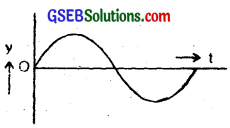The amplitude and frequency of these waves are same i.e. 3.0 cm and $$\frac{36}{2π}$$s-1 respectively.

Question 10.
For the travelling harmonic wave
y(x, t) = 2.0 cos 2π (10t – 0.0080x + 035)
where x and y are in cm and t in s. Calculate the phase difference between oscillatory motion of two points separated by a distance of (a) 4 cm, (b) 0.5 m (c) $$\frac{λ}{2}$$ (d) $$\frac{3λ}{4}$$.
Given equation of a travelling harmonic wave is
y(x, t) = 2.0 cos 2π(10t – 0.0080x + 0.35) ………………………… (1)
The standard equation of a travelling harmonic wave is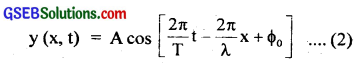Comparing equation (1) and (2), we get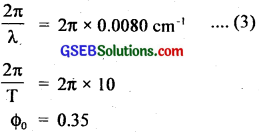We know that phase difference = $$\frac{2π}{λ}$$ × path difference …………………… (4)

(a) When path difference = 4m = 400 cm, then from (4)
phase difference = $$\frac{2π}{λ}$$ × 400
= 2π × 0.0080 × 40 [by using (3)]

(b) When path difference = 0,5m = 50 cm, then from (4)
phase difference = 2π × 0.0080 × 50

(c) When path difference = $$\frac{π}{2}$$, then
phase difference = $$\frac{2π}{λ}$$ × $$\frac{π}{2}$$ = π radian

(d) When path difference = $$\frac{3λ}{4}$$
phase difference = $$\frac{2π}{λ}$$ × $$\frac{3λ}{4}$$ = $$\frac{3π}{2}$$ rad = (π + $$\frac{π}{2}$$)
∴ cos (π + θ) = – cos θ
∴ effective phase difference = $$\frac{π}{2}$$.Question 11.
The transverse displacement of a string (damped at its both ends) is given by y(x, t) = 0.06 sin ($$\frac{2πx}{3}$$) cos (120πt) where x and y are in m and t in s. The length of the string is 1.5 m and its mass is 3.0 × 10-2 kg.
(a) Does the function represent a travelling or a stationary wave?
(b) Interpret wave as a superposition of two waves travelling in opposite directions. What are the wavelength, frequency and speed of each wave?
(c) Determine the tension in the string?
The given function is
y(x, t) = 0.60 sin ($$\frac{2πx}{3}$$) cos (120πt) ……………………. (i)
The travelling wave is represented by
y(x, t) = A sin $$\frac{2π}{λ}$$ (vt – x) …………………….. (ii)
and the stationary wave is represented by
y(x, t) = – 2A sin ($$\frac{2πx}{λ}$$) cos ($$\frac{2πvt}{λ}$$) ……………………….. (iii)

(a) Since the given function is similar to that of the function representing stationary wave, therefore, the given function represents a stationary wave (which involves harmonic functions of x and t separately).

(b) We know that when a wave
y1 = A sin $$\frac{2π}{λ}$$ (vt – x)
travelling along positive direction of x-axis is superposed by the reflected wave
y2 = – A sin $$\frac{2π}{λ}$$ (vt + x)
travelling in the opposite direction, a stationary wave
y = y1 + y2
= – 2A sin($$\frac{2π}{λ}$$x) cos ($$\frac{2π}{λ}$$ vt) …………………….. (iv)
is formed comparing equation (i) and (iv), we find that
$$\frac{2π}{λ}$$ = $$\frac{2π}{3}$$
or λ = 3 m
or $$\frac{2π}{λ}$$v = 120π
or v = 60λ = 60 × 3 = 180 ms-1
∴ frequency v = $$\frac{v}{λ}$$ = $$\frac{180}{3}$$ = 60 Hz
Velocity of transverse wave is given by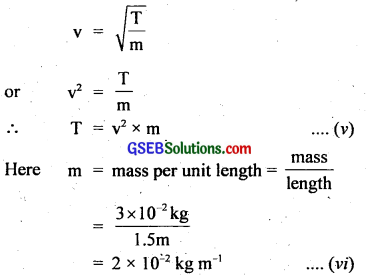v = 180 ms-1 of each wave.
T = tension in the string = ?

(c) ∴ From (v) and (vi), we get
T = (180)2 × (2 × 10-2)
= 32400 × 2 × 10-2 = 648 N.

Question 12.
(i) For the wave on a string described in exercise 15.11, do all the points on the string oscillate with the same (a) frequency, (b) phase, (c) amplitude? Explain your answers.
(ii) What is the amplitude of a point 0.375 m away from one end?
(i) All the points except nodes on the string have the same phase and frequency of oscillation but the amplitude is not same. This is because of the fact that the equation y (x, t) 0.06 sin ($$\frac{2π}{3}$$ x) cos (120πt) represents a stationary wave in which different points have different amplitude including zero at node to a certain maximum amplitude at antinode. But time period or frequency of oscillation of the particles have same value.

(ii) The given equation is
y(x, t) = 0.06 sin ($$\frac{2π}{3}$$ x) cos (120πt)
The amplitude of this equation i.e. of the wave represented by it is given by
A = 0.06 sin ($$\frac{2π}{3}$$ x)
At x = 0.375m
A = 0.06 sin ($$\frac{2π}{3}$$ × 0.375)
= 0.06 × sin (250π)
= 0.06 sin ($$\frac{π}{4}$$)
= 0.06 × $$\frac{1}{\sqrt{2}}$$
= 0.06 $$\frac{\sqrt{2}}{2}$$ = 03 $$\sqrt{2}$$
= 0.03 × 1.414
= 0.04242 m
= 0.042 m.Question 13.
Given below are some functions of x and t to represent the displacement (transverse or longitudinal) of an elastic wave. State which of these represent (i) a travelling wave, (ii) a stationary wave or (iii) none at all:

1. y = 2cos (3x) sin (10t)
2. y = 2$$\sqrt{x-vt}$$
3. y = 3 sin (5x – 0.5t) + 4 cos (5x – 0.5t)
4. y – cos x sin t + cos 2x sin 2t.

1. This equation has two harmonic functions of each x and t separately, so it represents stationary wave.
2. This function does not represent any wave as it contains no harmonic function.
3. It represents progressive/travelling harmonic wave as the arguments of cosine and sin functions are same.
4. This equation is the sum of two functions cos x sin t and cos 2x sin 2t each representing a stationary wave. Therefore it represents superposition of two stationary waves.

Question 14.
A wire stretched between two-rigid supports vibrates in its fundamental mode with a frequency of 45 Hz. The mass of the wire is 3.5 × 10-2 kg and its linear density is 4.0 × 10-2 kgm-1. What is (a) the speed of a transverse wave in the string and (b) the tension in the string?
Here, Frequency, v = 45 Hz
Mass of wire, M = 3.5 × 10-2 kg
m = mass per unit length
= linear density = 4.0 × 10-2 kg m-1
If L be the length of the wire or string, then
from formula, m = $$\frac{M}{L}$$, we get
L = $$\frac{M}{m}$$ = $$\frac{3.5 \times 10^{-2}}{4 \times 10^{-2}}$$ = 0.875 m
v = ?, T = Tension in the string = ?

(a) We know that the frequency of fundamental mode of the vibrating string is given by
(∴ in fundamental mode of vibration, L = $$\frac{λ}{2}$$ or λ = 2L)
v = $$\frac{v}{λ}$$ = $$\frac{v}{2L}$$
or v = v × 2L
= 45 × 2 × 0.875 = 78.75 ms-1
= 79 ms-1
where v = speed of transverse wave.

(b) Also we know that v = $$\sqrt{\frac{\mathrm{T}}{\mathrm{m}}}$$ = speed of transverse wave.
∴ T = v2 × m
= (78.5)2 × 4 × 10-2 = 248.06 N.Question 15.
A metre-long tube open at one end, with a movable poston at the other end, shows resonance with a fixed frequency source (a tuning fork of frequency 340 Hz) when the tube length is 25.5 cm or 79.3 cm. Estimate the speed of sound in air at the temperature of the experiment. The edge effects may be neglected.
The tube with a piston makes a closed organ pipe.
Let l1 and l2 be the resonance lengths for nth and (n + 1) the modes of vibration of the closed pipe respectively.
∴ l1 = 25.5 cm
l2 = 79.3 cm (given)
If v be the velocity of sound wave, then frequency v1 and v2 for these modes are given by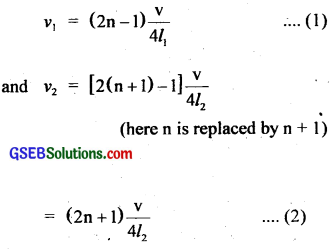Both the modes are in resonance with a frequency of 340 Hz.
∴ v1 = v2 = 340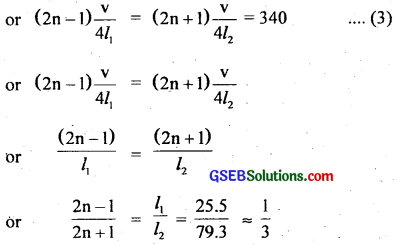(Approximation has been used because edge effect is being ignored. Also in closed organ pipe second resonance length is 3 times the first resonance length)
or 3(2n – 1) = 2n + 1
or 6n – 3 = 2n ± 1
or 6n – 2n = 3 + 1
or 4n = 4
∴ n = 1
Putting n = 1, in equation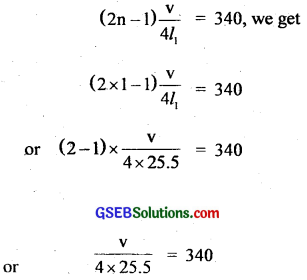or v = 340 × 4 × 25.5
or v = 340 × 102 = 34680 cms-1
= 346.8 ms-1 = 347 ms-1.

Question 16.
A steel rod 100 cm long is clamped at its middle. The fundamental frequency of longitudinal vibrations of the rod are given to be 2.53 kHz. What is the speed of sound in steel?
A rod clamped in the middle has antinodes (A) at its ends and node (N) at the point of clamping. In fundamental mode, thus the length of the rod is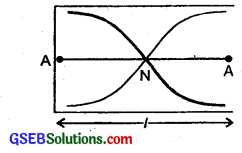l = $$\frac{λ}{2}$$ or λ = 2l
where l = length or rod
and λ = wavelength of the wave
Here, l = 100 cm
v = 2.53 kHz = 2.53 × 103 Hz
∴ λ = 2 × 100 = 200 cm
If v be the speed of sound in steel, then
v = vλ
= 2.53 × 103 × 200
= 506 × 103 cms-1
= 5.06 × 103 ms-1
∴ v = 5.06 kms-1.Question 17.
A pipe 20 cm long is closed at one end. Which harmonic mode of the pipe is resonantly excited by a 430 Hz source? Will the same source be in resonance with the pipe if both ends are Open? (speed of sound in air is 340 ms-1).
Here, l = 20 cm = 0.20 m
v = 340 ms-1
frequency of exciting source, vn = 430 Hz
We know that the frequency of nth mode of vibration of a closed pipe is given by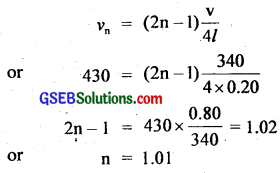or n = 1.01
i.e. the organ pipe is in the first harmonic or fundamental mode of vibration.
In case of an open pipe, frequency of nth mode of vibration is given by
vn = n $$\frac{v}{2l}$$
where length l in fundamental mode = $$\frac{λ}{2}$$ or λ = 2l
or 430 = $$\frac{n×340}{2×0.2}$$
or n = $$\frac{430×0.4}{340}$$ = $$\frac{172}{340}$$ ≈ 0.5
As, n has to be an integer i.e. can have integer values, so n = 0.5 is not valid. Hence the same source cannot be in resonance with the open pipe.

Aliter:
Here, the fundamental frequency for closed end pipe is:
v = $$\frac{v}{4l}$$ = $$\frac{340}{4×0.2}$$ = 425 Hz
This is the first harmonic. The second harmonic is
3v = 3 × 425 = 1275 Hz
3rd harmonic is 5v = 5 × 425 = 2125 Hz. etc.
∴ Only the 1st harmonic of frequency 425 Hz is resonantly excited by a 430 Hz source.
Now let v’ = fundamental frequency of pipe open at both ends.
∴ v’ = $$\frac{v}{2l}$$ = $$\frac{340}{2×0.2}$$ = 850 Hz.
2nd, 3rd, 4th etc. harmonics have frequencies 2v’, 3v’, 4v’ …………………. = 1700 Hz, 2550 Hz ………………… etc.
∴ No harmonic can be excited by the 430 Hz source in this case.

Question 18.
Two sitar strings A. and B playing the note ‘Ga’ are slightly out of tune and produce beats of frequency 6 Hz. The tension in the string A is slightly reduced and the beat frequency is found to reduce to 3 Hz. If the original frequency of A is 324 Hz, what is the frequency of B?
We know that v ∝ $$\sqrt{T}$$ where v = frequency, T = tension
The decrease in the tension of a string decreases its frequency. So let us assume that original frequency vA of A is more than the frequency vB of B.
Thus vA – vB = ±6 Hz (given)
and vA = 324 Hz
∴ 324 – vB = ±6
or vB = 324 ± 6 = 318 Hz or 330 Hz.
On reducing tension of A, ∆v = 3 Hz
If vB = 330 Hz and on decreasing tension in A, vA will be reduced i.e. no. of beats will increase, but this is not so because no. of beats becomes 3.
∴ vB must be 318 Hz because on reducing the tension in string A, its frequency may be reduced to 321 Hz, thus giving 3 beats with vB = 318 Hz.Question 19.
Explain why (or how):
(a) in a sound wave, a displacement node is a pressure antinode and vice versa,
(b) bats can ascertain distances, directions, nature and sizes of the obstacles without any‘eyes’,
(c) a violin note and sitar note may have the same frequency, yet we can distinguish between the two notes,
(d) solids can support both longitudinal and transverse wave but only longitudinal waves can propagate in gases, and
(e) the shape of pulse gets distorted during propagation in a dispersive medium.
(a) In a sound wave, a node is a point where the amplitude, of oscillation i.e. displacement is zero as here a compression and a rarefaction meet and the pressure is maximum, so it is called pressure antinode. While an antinode is a point where the amplitude of oscillation is maximum i.e. displacement is maximum but pressure is minimum. So this point is called pressure node., Hence displacement node coincides with pressure antinode and displacement antinode with pressure node.

(b) Bats emit ultrasonic waves of large frequencies (small wavelength) when they fly. These ultrasonic waves are received by them after reflection from the obstacle. Their ears are so sensitive and trained that they not only get the information of the distance of the obstacle but also that of the nature of the reflecting surface.

(c) The quality of the sound produced by an instrument depends upon the number of overtones. Since the number of overtones is different in the cases of sounds produced by violin and sitar therefore we can distinguish the notes produced by them.

(d) This is because solids have both the elasticity of volume and elasticity of shape (called shear elasticity), so both longitudinal and transverse waves can propagate through them. On the other hand, gases have only the volume elasticity and no shear elasticity, so only longitudinal waves can propagate in gases.

(e) A sound pulse is a combination of waves of different wavelengths. In a dispersive medium, the waves of different wavelengths travel with different speeds in different directions i.e. with different velocities. So the shape of the pulse gets distorted i.e a plane wavefront in a non-dispersive medium does not remain a plane wavefront in a dispersive medium.Question 20.
A train standing at the outer signal of a railway station blows a whistle of frequency 400 Hz in still air.
(i) What is the frequency of the whistle for a platform observer when the train (a) approaches the platform with a speed of 10 ms-1, (b) recedes from the platform with a speed of 10 ms-1.
(ii) What is the speed of sound in each case? The speed of sound in still air can be taken as 340 ms-1.
Here, frequency of source of sound,
v = 400 Hz
v = 340 ms-1
= speed of sound
speed of source = vs = 10 ms-1

(i) (a) When the train approaches the platform, the apparent frequency as heard by the observer on the platform will be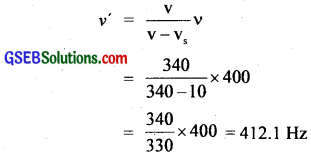(b) When the train recedes from the platform, the apparent frequency as heard.by the observer is given by according to the formula:
v’ = $$\frac{\mathbf{V}}{\mathbf{v}-\mathbf{v}_{\mathbf{s}}}$$
= $$\frac{340}{340+10}$$ × 400
= $$\frac{340}{350}$$ × 400 = 388.6 Hz = 389 Hz

(ii) The speed of sound in each case remains same i.e. 340 ms-1.Question 21.
A train standing in a station yard blows a whistle of frequency 400 Hz in still air. A wind starts blowing in the direction from the yard to the station with a speed of 10 ms-1. What are the frequency, wavelength and speed of sound for an observer standing on the station’s platform? Is the situation exactly identical to the case when the air is still and the observer runs towards the yard at a speed of 10 ms-1? The speed of sound in still air can be taken as 340 ms-1.
Here, v = 400 Hz
v = 340 ms-1

(a) When wind blows listener at rest:
Speed of wind, w = 10 ms-1
Now for a stationary observer on the platform, the frequency does not change as there is no relative motion between him . and the source at rest.
∴ Frequency of sound received by observer v’= v = 400 Hz
As the wind is blowing in the same direction in which sound waves are moving, therefore apparent velocity of sound (v’) is
= speed of sound + speed of wind
or v’ = v + w
= 340 + 10
= 350 ms-1
∴ wavelength of sound for stationary listener,
λ’ = $$\frac{v’}{v’}$$ = $$\frac{350}{340}$$ = 0.875 m

(b) When listener moves towards stationary source i.e. train and wind at rest:
speed of observer, v0 = 10 ms-1
As the listener (observer) runs towards the train (source at rest, so apparent frequency (v”) is given by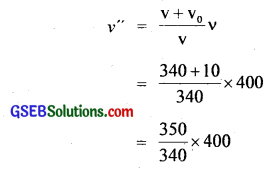or v” = 411.8 Hz = 412 Hz.
Speed of sound relative to the listener in motion is given by:
v” = v – v0
= 340 – 10 = 330 ms-1
In this case wavelength of sound will remain unchanged. Thus we conclude that the situations (a) and (b) are not exactly identical.